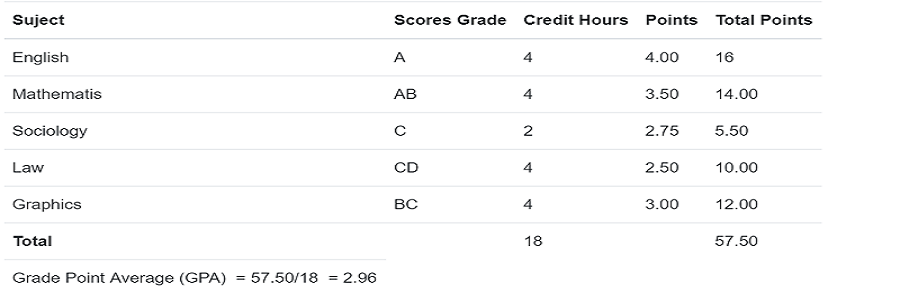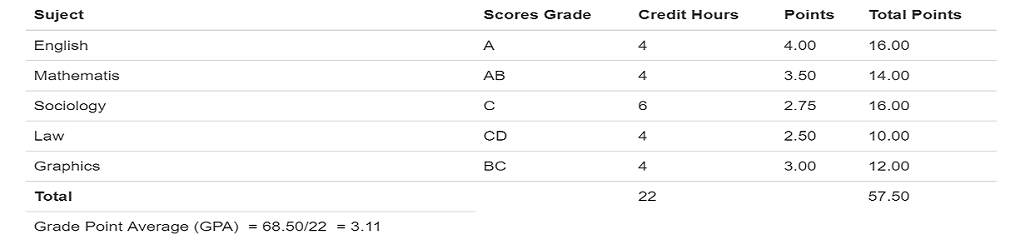# ACADEMIC POLICY AND REGULATIONS

## ACADEMIC POLICY AND REGULATIONS

The academic policy of the Federal Polytechnic, Damaturu, is based on the existing standards of the National Board for Technical Education (NBTE) which aims at achieving uniformity in all the polytechnics in the country.
The form of curriculum organization whereby the Work syllabi in a particular programme of studies are divided into courses in prescribed sections of teaching and study, each of which is separately examined and assessed is in practice.

#### Course Credit System

The course credit system seeks to avoid package repetition of instructional components earlier assessed as passed, and which may on. The other hand improve performance in the instructional components earlier assessed as failed. This will provide specification in the workloads by enabling more precise specification of the different required components and the ancillary courses of various instructional programme.

#### Definition of Basic Terms

1. Session means an academic year as determined by the Academic Board which shall normally be of two semesters.
2. Semester means period of about 18 weeks including 15 contact weeks.
3. Contact Hour is defined as the period of classroom, laboratory, workshop or fieldwork interaction between a tutor and students for academic instruction supervision.
4. One Credit is defined as one hour of lecture or tutorial per week per semester or two-three hours of field, laboratory, studio or workshop practical per week per semester.
5. Compulsory Courses are courses that must be taken and passed in particular programme of studies.
6. Elective Courses are, optional courses that once chosen would have to be passed.
7. Examination means a formal written, spoken or practical test.
8. Grade Point (GP) is derived from the actual percentage raw score given score: the score is then converted into a letter grade and a grade point.
9. Grade Point Average (GPA) is the ratio of the total Grade Points (GP) to the total number of semester hours. Credit points are calculated by multiplying the course grade point by the semester hour value of the course.
10. Cumulative Grade Point Average (CGPA) is the ratio of the total of the credit points for the present and previous semesters to the total numbers of semester hours for the present and previous semesters. In other words, it is the up-to-date mean of the grade points earned by the student in a programme of study which in effect indicates the student’s overall performance at any point in the training programme.

### METHODS FOR COMPUTATION AND ILLUSTRATION OF STUDENTS OVERALL PERFORMANCE

#### Grade Point (GP)

Grade Point is obtained by multiplying the credit hours assigned to a particular course by hours point obtained in the examination of that course. For example if property law carries 4 credits and a student obtained 80%, which is given the grade A and which is 4 points, his grade point for property Law will be 4x4=1 6.

#### Grade Point Average (GPA)

The overall performance of each student shall be determined by means of grade point average obtained by awarding credit points in respect of each course on the basis of semester hour of the course multiplied by the Numerical value of the grade obtained. The grade point average is then the total number of Semester hours.

For example, if a student Scores A(75%), BC(60%),B(65%), C(55%) and E(40%) in five subjects which carry student’s cumulative grade point average. it is simply a 3, 2, 1, 3, and 3 credit hours respectively his/her grade weighted average. For example if a student’s scores for point average will be worked out as follows.If the credit hour attached to the subject were to change higher/lower, the student’s grade point will move higher or lower. This emphasizes the need for a student to work hard and score highly in all, but particularly in courses with the highest credit hours, since this will step up his/her grade point average, while a low score in the highest credit course will cause sharp drop in his/her grade point average. Since this will step .up his/her grade point average, while a low score in the highest credit subjects will cause a sharp drop in his/her grade point average.

#### Cumulative Grade Point Average (CGPA)

The calculation of a grade point average obtained by a student in two or more semesters is referred to as the student’s cumulative grade point average. It is simply a weighted average. For example if student’s scores for two semesters were as follows:

1st SEMESTER2nd SEMESTERCumulative Points Average = 1st semester GPA+ 2nd Semester GPA = (3.19+3.11)/2 = (6.30)/2 =3 15